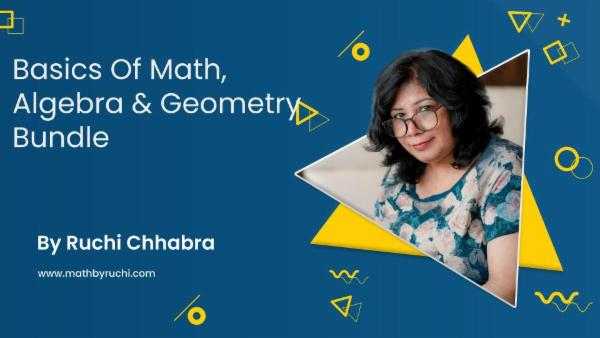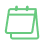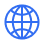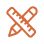### Basics of Math, Algebra & Geometry Bundle

Builds a strong foundation in Pre-Algebra, Algebra 1, Algebra 2 & Geometry for school & competitive exams

Language: English

# About the package

Description

There are three courses in this bundle

1) Perfect Your Math Fundamentals (Pre-Algebra)

2) Perfect Your Algebra Fundamentals

3) Geometry from basics to advanced (Part 1)

Who is this Bundle for?

-Current IGCSE, CBSE & ICSE students

-Middle school, High school, or early college level students

-Those studying for competitive exams and wants to revise their basics

-Anyone who wants to brush up on the basics of Math, Algebra & Geometry

-Parents looking for extra support with the Fundamentals of Mathematics

- GMAT/ GRE/MBA/Olympiads/NTSE entrance exams students looking for a revision of Math fundamentals

What are the requirements or perquisites for taking this Bundle?

-Knowledge of Math up to 5th grade

What will you learn?

-Lines & Angles, Complementary & Supplementary Angles, Parallel lines, Polygons, Triangles, Congruent Triangles, Inequalities in a Triangle, Pythagorean Theorem, Quadrilaterals, Types of Trapeziums, Parallelograms, Rectangles, Rhombus, Squares, Kites

-Number System, Integers, Fractions & Decimals and their operations, Factors & Multiples, PEMDAS, BODMAS, Ratio & Direct and Inverse Proportion, Rational Numbers, Irrational Numbers, Rounding off Numbers, Estimation and approximation, Percentage, Profit & Loss, Discounts & Taxes Topics

-Algebra from scratch, Terms, Coefficients, Degree, Addition, Subtraction, Multiplication & Division of algebraic expressions, Numerical Value of Algebraic Expressions, BODMAS, Higher Exponent, subject of the formula, Algebraic Fractions to its lowest form, Graphs, Linear equations in one variable, Linear Inequalities, Simultaneous linear equations, Quadratic equations

-Quadratic polynomials, Zeros of polynomials α, β & γ, Remainder Theorem & Factor Theorem, Factorization Techniques like common factors, regrouping, splitting the middle term and using identities & Algebraic Identities like

(a - b) ², (a + b) ³, a ³ - b ³, (a + b + c) ² etc.

# What you’ll learn-Lines & Angles, Complementary & Supplementary Angles, Parallel lines, Polygons, Triangles, Congruent Triangles, Inequalities in a Triangle, Pythagorean Theorem, Quadrilaterals, Types of Trapeziums, Parallelograms, Rectangles, Rhombus, Squares, Kites-Number System, Integers, Fractions & Decimals and their operations, Factors & Multiples, PEMDAS, BODMAS, Ratio & Direct and Inverse Proportion, Rational Numbers, Irrational Numbers, Rounding off Numbers, Estimation and approximation, Percentage, Profit & Loss, Discounts & Taxes Topics-Algebra from scratch, Terms, Coefficients, Degree, Addition, Subtraction, Multiplication & Division of algebraic expressions, Numerical Value of Algebraic Expressions, BODMAS, Higher Exponent, subject of the formula, Algebraic Fractions to its lowest form, Graphs, Linear equations in one variable, Linear Inequalities, Simultaneous linear equations, Quadratic equations-Quadratic polynomials, Zeros of polynomials α, β & γ, Remainder Theorem & Factor Theorem, Factorization Techniques like common factors, regrouping, splitting the middle term and using identities & Algebraic Identities like

(a - b) ², (a + b) ³, a ³ - b ³, (a + b + c) ² etc.

# Reviews and TestimonialsLaunch your Graphy
100K+ creators trust Graphy to teach online